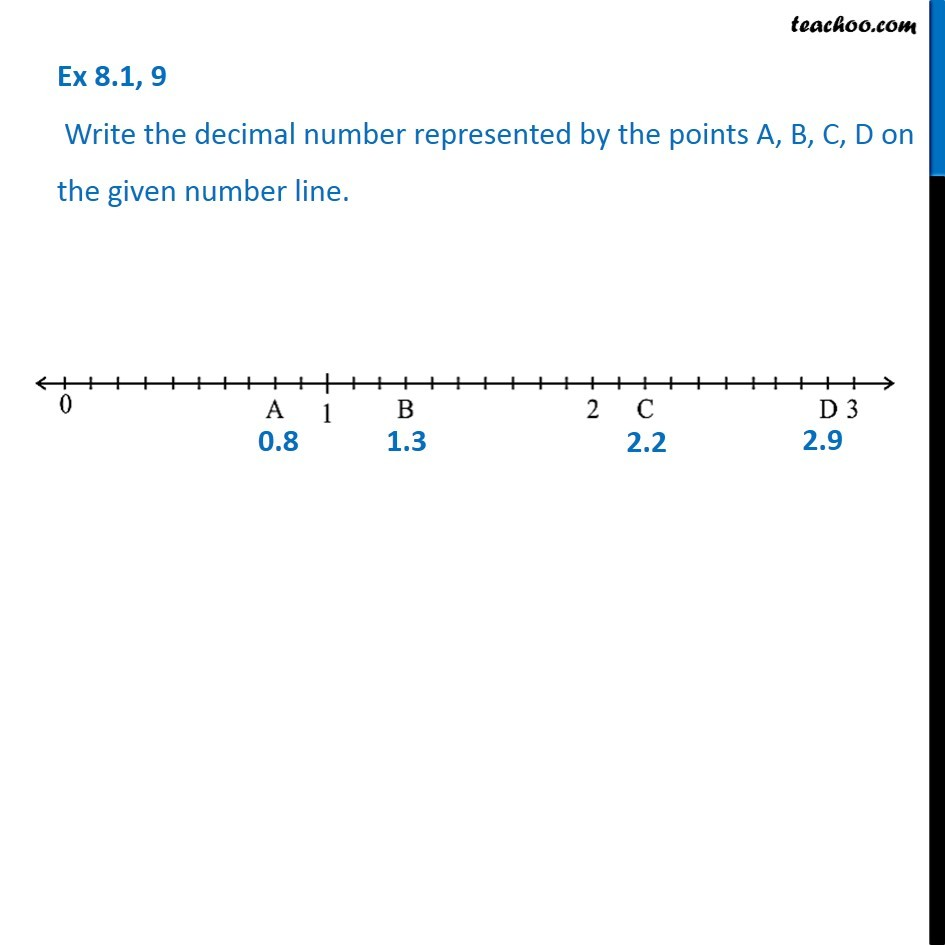Subscribe to our Youtube Channel - https://you.tube/teachoo

1. Chapter 8 Class 6 Decimals
2. Concept wise
3. Decimals in number line

Transcript

Ex 8.1, 9 - Chapter 8 Class 6 Decimals - NCERT Solution Write the decimal number represented by the points A, B, C, D on the given number line. From the number line, we mark number line as 0.1, 0.2, 0.3, and so on So, our points are A = 0.8 B = 1.3 C = 2.2 D = 2.9

Decimals in number line